Worksheet - Jugs And Mugs

# Worksheet - Jugs And Mugs | Mathematics for Class 4: NCERT

 1 Crore+ students have signed up on EduRev. Have you?

Q.1. A shopkeeper uses 20 ml of milk to make one cup of tea; if he sell 50 cups of tea then how much milk did he use?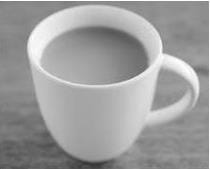Ans.
1000 ml

Q.2. Fill in the blanks:
(i) 300 ml + _______ = 1 Litre
(ii) 500 ml + _______ = 1 Litre
(iii) 450 ml + _______ = 1 Litre
Ans.

(i) 300 ml + 700 ml = 1 litre
(ii) 500 ml + 500 ml = 1 litre
(iii) 450 ml + 550 ml = 1 litre

Q.3. How many 250 ml of Glasses we need to serve 1000 ml of soup?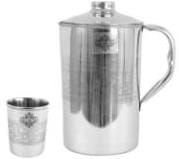Ans.
4

Q.4. Estimate the capacity of a table spoon (Tick the right answer)
(a) 15 ml
(b) 1500 ml
(c) 150 ml
(d) 1.5 ml
Ans.
(a)

Q.5. Arrange the following objects in order of increasing capacity. Write numbers 1.2.3 under them: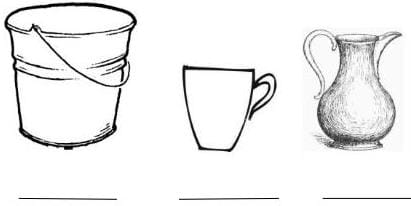Ans.
1, 3, 2

Q.6. Match the following:

 (a) 6 ml + 4 ml + 5 ml ½ Litre (b) 350 ml + 150 ml 750 ml (c) 650 ml + 350 ml 2 ml + 8 ml + 5 ml (d) 400 ml + 100 ml + 250 ml 1 Litre

Ans.

 (a) 6 ml + 4 ml + 5 ml 2 ml + 8 ml + 5 ml (b) 350 ml + 150 ml ½ Litre (c) 650 ml + 350 ml 1 Litre (d) 400 ml + 100 ml + 250 ml 750 ml

Q.7. You are trying to pour tea in 50 ml cups from a 250 ml cup, but you spilled 25 ml while doing it. what is amount of tea in 5th cup?
Ans.
25 ml

Q.8. A tea shop need 5 litres of milk every day. Each litre costs ₹ 40. How much money is needed to buy milk for 5 days?
Ans.
₹ 1,000

Q.9. Neetu has to take 3 injections in a day for 5 days. If one injection gives her 3 ml of medicine, then how much medicine she is getting every day?
Ans.
9 ml

Q.10. Preksha has 1 litre of water in her bottle. Four of her friends are thirsty so she equally distributed water among them. How much water will be there in each bottle, mark.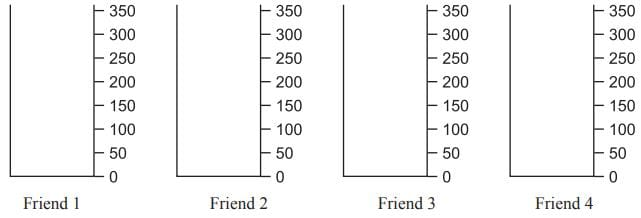Ans. Each container will have 250 ml of water.

Q.11. How many milliliters do we have in 3.5 liter?
Ans.
3500 ml

Q.12. Draw :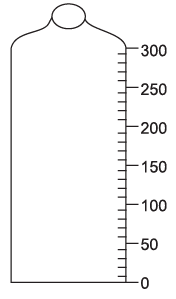Aman's sister drinks 270 ml of milk in the morning. His mother has gone out for work today so he has to prepare milk. Draw the mark upto which the milk will be poured  by him.
Ans.
Children marke it themselves.

Q.13. Amina's water bottle holds one liter of water. She drank 250 ml of water and her friend Govind drank 150 ml. How much water is left in her bottle?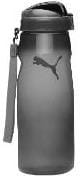Ans.
600 mL

Q.14. Study the data and answer :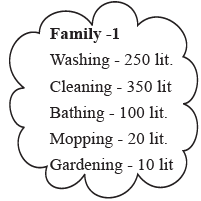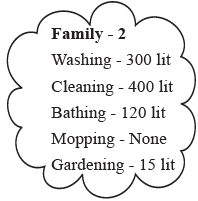(i) Which family uses less water judiciously?
(ii) How much total water is used by each family?
Ans.
(a) Family 1

Q.15. what capacity can be measured by given items, l or ml?
(a)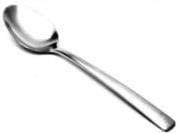(b)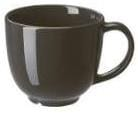Ans.

(a) ml
(b) ml

Q.16. Which is more:
1 Litre or 1000 ml
_____________
Ans.
1 liter = 1,000 ml
Both are equal.

Q.17. Which one of the following has more capacity?
(a)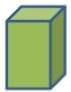(b)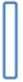Ans.
(a)

Q.18. 1/2 Litre is equal to
(a) 200 ml
(b) 500 ml
(c) 100 ml
(d) 700 ml
Ans.
1/2 litre = 500 ml

Q.19. How many liters you would get if you add 1200 ml and 1800 ml?
Ans.
31

Q.20. Encircle the wrong combination: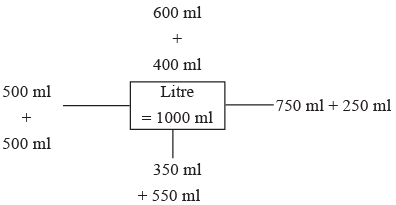Ans.

350 ml + 550 ml is the wrong combination
350 ml + 550 ml = 900 ml
1 lit. = 1,000 ml. Hence it is wrong.

The document Worksheet - Jugs And Mugs | Mathematics for Class 4: NCERT is a part of the Class 4 Course Mathematics for Class 4: NCERT.
All you need of Class 4 at this link: Class 4

## Mathematics for Class 4: NCERT

24 videos|44 docs|32 tests

## Mathematics for Class 4: NCERT

24 videos|44 docs|32 tests

Track your progress, build streaks, highlight & save important lessons and more!(Scan QR code)

,

,

,

,

,

,

,

,

,

,

,

,

,

,

,

,

,

,

,

,

,

;